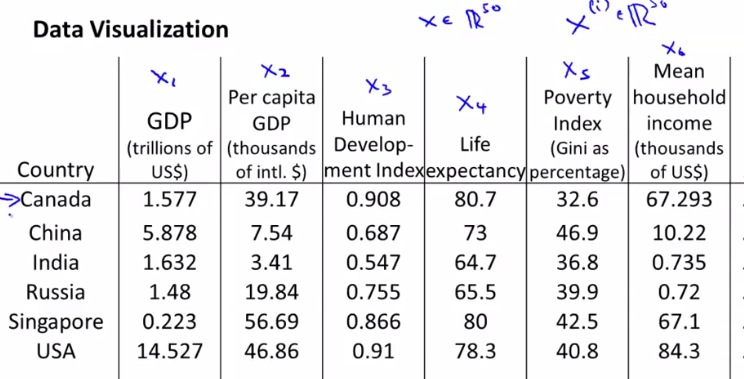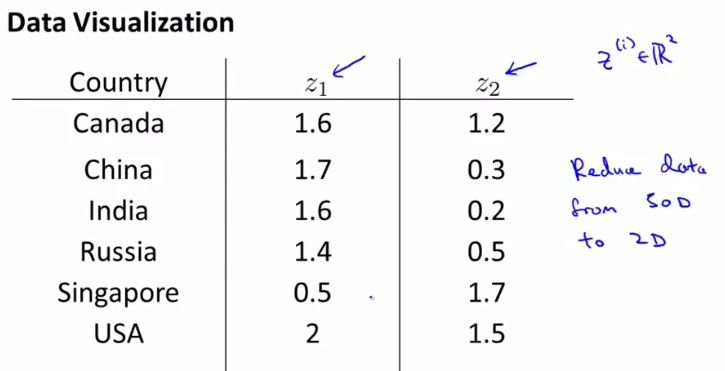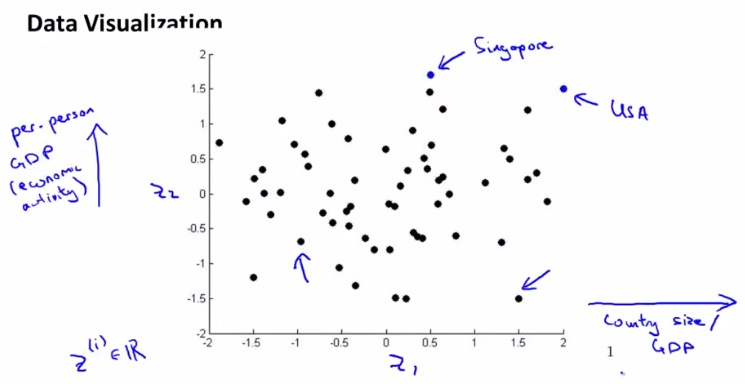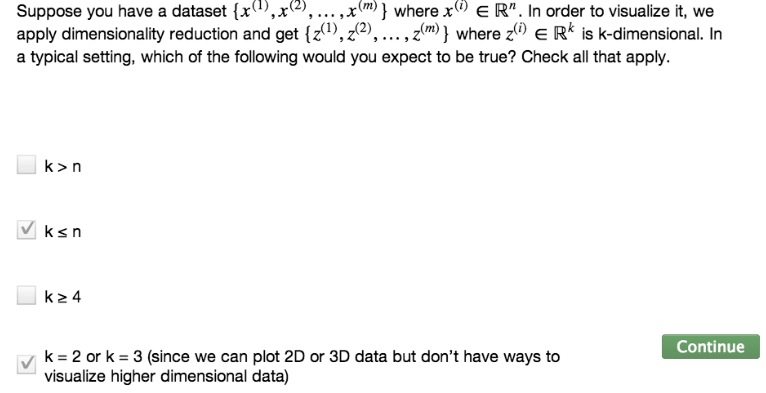• Last: DR for compressing data
• Here DR for visualize data
• DV makes better understanding of the data• Huge graph data that maybe contain 50 parameter
• Difficult to visualize  make 50-D graph,
• Instead represent each vector, represent countriy in R2.
• Makes parameters into one vector. Somehow find the correlation and makes 50-D input to 2D input• As example z1 = country size. Generalized for GDP countries
• z2 = per person GDP
• Example Singapore (bottom right, high per person, but small countries)
• USA : per person high, countries: large
• Find a correlation that takes 2D graph to represent the data (Example here, to generalize it as finding the economic activity and its correlation between each country and people that reside within it• This is how to make 2D graph representation. It's important to make it 2D to make us better seeing the data as a whole.This makes us understand better about what data trying to give us, and makes appropriate action based on the graph.
•• PC analysis, doing DR, and how to compress data